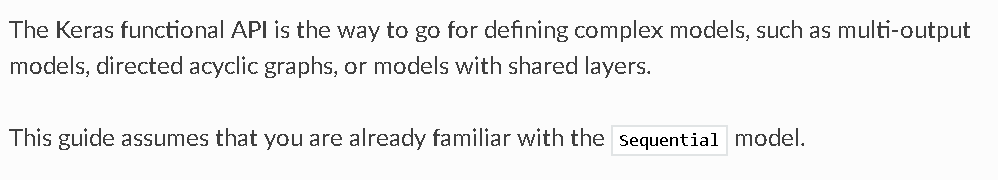# keras Model 1 入门篇

1 入门

2 多个输入和输出

3 共享层

1、 序列模型  The Sequential model

2、 函数式模型  the Keras functional1、 每个layer实例都是可以调用的，它返回一个tensor

2、 输入tensor和输出tensor可以用来定义一个Model

3、 函数式的模型也可以像 Sequential模型一样训练。

from keras.layers import Input, Dense
from keras.models import Model

# This returns a tensor
inputs = Input(shape=(784,))

# a layer instance is callable on a tensor, and returns a tensor
x = Dense(64, activation='relu')(inputs)
x = Dense(64, activation='relu')(x)
predictions = Dense(10, activation='softmax')(x)

# This creates a model that includes
# the Input layer and three Dense layers
model = Model(inputs=inputs, outputs=predictions)
model.compile(optimizer='rmsprop',
loss='categorical_crossentropy',
metrics=['accuracy'])
model.fit(data, labels)  # starts training

x = Input(shape=(784,))
# This works, and returns the 10-way softmax we defined above.
y = model(x)

from keras.layers import TimeDistributed

# Input tensor for sequences of 20 timesteps,
# each containing a 784-dimensional vector
input_sequences = Input(shape=(20, 784))

# This applies our previous model to every timestep in the input sequences.
# the output of the previous model was a 10-way softmax,
# so the output of the layer below will be a sequence of 20 vectors of size 10.
processed_sequences = TimeDistributed(model)(input_sequences)

posted @ 2019-06-04 12:54  超级学渣渣  阅读(...)  评论(...编辑  收藏